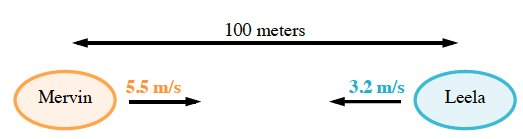### Home > GC > Chapter 5 > Lesson 5.1.3 > Problem5-27

5-27.

Mervin and Leela are in bumper cars. They are at opposite ends of a $100$-meter track heading toward each other. If Mervin moves at a rate of $5.5$ meters per second and Leela moves at a rate of $3.2$ meters per second, how long does it take for them to collide?Let $p=\text{position (m)}$and $t = \text{time (sec)}$

Remember Mervin is starting at $0$ because he is on the far left. His growth rate is $5.5$ m/s to the right.

So the equation for Mervin's position is $p=5.5t+0$, but the $0$ is not needed so $p=5.5t$ is sufficient.

Do the same for Leela. Remember that her growth is negative and her starting position is different

Since Mervin and Leela will meet in the same position, set $\text{Mervin's position}=\text{Leela's position}$. This is like solving a system of equations using the equal values method.